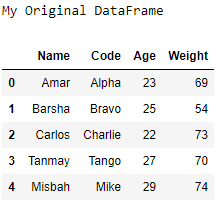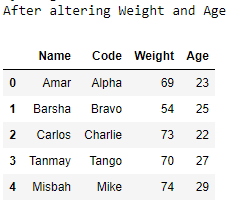Change the order of a Pandas DataFrame columns in Python

• Last Updated : 11 Aug, 2021

Let us see how to change the order of a DataFrame’s columns.

Algorithm :

• Create a DataFrame.
• Reassign the same DataFrame with the order of the columns changed.
• Print the DataFrame.

Example 1 :

Python3

 # importing the modulesimport pandas as pdimport numpy as np # creating the DataFramemy_data = {'Sr.no': [1, 2, 3, 4, 5],           'Name': ['Ram', 'Sham', 'Sonu',                    'Tinu', 'Monu'],           'Maths Score': [45, 67, 89, 74, 56]}df = pd.DataFrame(data = my_data) # printing the original DataFrameprint("My Original DataFrame")print(df) # altering the DataFramedf = df[['Sr.no', 'Maths Score', 'Name']] # printing the altered DataFrameprint('After altering Name and Maths Score')print(df)

Output:Example 2 :

Python3

 # importing the modulesimport pandas as pd  # creating the DataFramel1 =["Amar", "Barsha", "Carlos", "Tanmay", "Misbah"]l2 =["Alpha", "Bravo", "Charlie", "Tango", "Mike"]l3 =[23, 25, 22, 27, 29]l4 =[69, 54, 73, 70, 74]df = pd.DataFrame(list(zip(l1, l2, l3, l4)))df.columns =['Name', 'Code', 'Age', 'Weight'] # printing the original DataFrameprint("My Original DataFrame")print(df)  # altering the DataFramedf = df[['Name', 'Code', 'Weight', 'Age']]  # printing the altered DataFrameprint('After altering Weight and Age')print(df)

Output :My Personal Notes arrow_drop_up